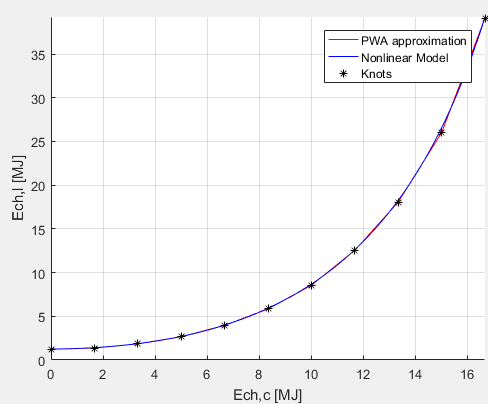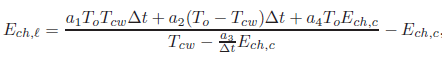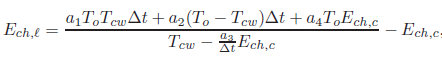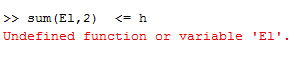# Disciplined convex programming error: Cannot perform the operation: {real affine} .* {convex}

Hi everyone. I’ve subscribed a week ago since I’ve been struggling with modelling problem ever since, but before resorting to your help I’ve tried to follow FAQ, DCP ruleset and so on but I’ve not been able to figure out the issue I got stuck at.
I’m working on a thesis project whose aim is to minimize a cost function representing the energy distribution cost among buildings in function thermal and energetic exchanges.

My code is as follows:

"a1=0.0109;
a2=20.22;
a3=3.8;
a4=0.9327
To=295 %outside temperature
Tcw=283 %cooling water temperature

x0(:,i) = inv(eye(Building.Parameters.ns)-(Building.Model.Ax))
(Building.Model.Bx
Tz(:,i)+Building.Model.Wx*Disturbances.Mean);

% Energy required for cooling;
Ec(:,i) = Building.Model.Asx0(:,i) +…
+ Building.Model.Bs
Tz(:,i) +…
+ Building.Model.Ws*Disturbances.Mean +…
+ Disturbances.Intenal +…
+ Disturbances.People + …

% Energy required to the chiller;
Ech(:,i) = Ec(:,i) - s(:,i);

% Electricity
El(:,i)=(a1ToTcwDt+a2(To-Tcw)Dt+a4To*Ech(:,i)).pow_p(Tcw-a3Ech(:,i)/Dt,-1)-Ech(:,i);"

where El(:,i) is a vector representing the elctrical energy consumption analyzed as function of the variable Ech (cooling energy in this case), whereas a1,a2,a3,a4 are fixed coefficients
Disciplined convex programming error:
Cannot perform the operation: {real affine} .* {convex}

Unfortunately every time I run this code, cvx gives me the expression:
“Disciplined convex programming error:
Cannot perform the operation: {real affine} .* {convex}”

Same statement if I write:
El(:,i)=(a1ToTcwDt+a2(To-Tcw)Dt+a4ToEch(:,i))./(Tcw-a3Ech(:,i)/Dt,-1)-Ech(:,i);

The point is that I’ve checked both graphically both mathematically the convexity of this expression, and my professor likewise told me that this expression is convex?
I’d be grateful if you all could help me out to find another way to write that expression in a proper way. I swear I’ve followed-as mentioned above-all the guidelines but still I’ve not been able to find a solution throughout the past week

Paolo De Bella

You haven’t told us what your CVX variables are.

How did you mathematically check the convexity of the expression?

Please use the “preformatted text” so that nothing will be lost in your formulas and they will be easier to read.

You’re right. The full code is:

``` m = 3;                   % Number of agents;
M = 144;                 % Number of time slots;
Dt = 600;                % Width of the time slot [s];
a1=0.0109;
a2=20.22;
a3=3.8;
a4=0.9327;
Echmax=0.05*Dt;
Tcw=283;
To=295;
S0 = 750;                % Storage initial condition [MJ];
Smax = 1425;        % Storage max capacity [MJ];
Smin = 75;        % Storage max capacity [MJ];
smax = 33;               % Storage max flow [MJ];
smin = -smax;            % Storage min flow [MJ];
Losses = 0.99;           % Percent of losses per hour;
ratio = 10;              % alpha = mean(beta)/ratio;
usebeta = 1;             % 1 = use beta, 0 = do not;
c = 0.0037;              % Proximal coefficient;
cTz = 0.01;              % Penalization term on Temperatures;
cs = 0.0001;             % Penalization term on Storage;
deltabar = 1e-6;         % Dual ascent gain;
gamma = 0.1;             % Dual ascent vanishing parameter;
epsilonRo = 1e-3;        % Relative convergence tollerance outer layer;
epsilonAo = 1e-3;        % Absolute convergence tollerance outer layer;
epsilonRi = 1e-1;        % Relative convergence tollerance inner layer;
epsilonAi = 1e-1;        % Absolute convergence tollerance inner layer;
%------------- Optimization -----------------------------------------------

cvx_begin
cvx_quiet(true)

%-------- Optimization variables ------------------------------------------

variable h(M,1);                  % Epigraphic reformulation;
variable Tz(M+1,m);               % Temperatures;
variable s(M,m);                  % Storage flow;

dual variable y0;                 % To check tight;
dual variable y1;                 % Optimal multipliers;
dual variable y2;                 % Optimal multipliers;
dual variable y3;                 % Optimal multipliers;

cvx_precision high                % May cause failure

Penalization = 0;                 % Inizialization

for i = 1:m                       % For each building

% Stationary solution for the building state;
x0(:,i) = inv(eye(Building.Parameters.ns)-(Building.Model.Ax))*...
(Building.Model.Bx*Tz(:,i)+Building.Model.Wx*Disturbances.Mean);

% Energy required for cooling;
Ec(:,i)  = Building.Model.As*x0(:,i) +...
+ Building.Model.Bs*Tz(:,i) +...
+ Building.Model.Ws*Disturbances.Mean +...
+ Disturbances.Internal +...
+ Disturbances.People + ...

% Energy required to the chiller;
Ech(:,i) = Ec(:,i) - s(:,i);

% Electricity
El(:,i)=(a1*To*Tcw*Dt+a2*(To-Tcw)*Dt+a4*To*Ech(:,i)).*pow_p(Tcw-a3*Ech(:,i)/Dt,-1)-Ech(:,i);

% Penalization
Penalization = Penalization + ...
+ cTz*pow_pos(norm(Tz(:,i)-(273+23),2),2) + ...
+ cs*pow_pos(norm(s(:,i),2),2);
% "pow_pos(norm(x,2),2)" is better than "traspose(x)*x"
end

% Storage Energy content
S = Storage.Model.A*S0 + Storage.Model.B*sum(s,2);

% -------- Problem formulation ---------------------------------------------

minimize transpose(beta + alpha*h)*h + Penalization

subject to

for i = 1:m
% Comfort constraints
Tz(:,i)  <= Building.Constraints.Tzmax;
Tz(:,i)  >= Building.Constraints.Tzmin;
% Technical limits
Ech(:,i) <= Chiller{i}.Constraints.Echmax;
end
% Closure contraint - initial condition
Tz(1,1:i)  == Tz(end,1:i);
Tz(1,1:i)  == ones(1,m)*(273+24);
% Do not heat
Ec  >= 0;
Ech >= 0;
% Absorbed Electricity
y0:sum(El,2)  <= h;
% Storage Constraints
s         <= Storage.Constraints.smax/3;
s         >= Storage.Constraints.smin/3;
y1:S      >= Storage.Constraints.Smin;
y2:S      <= Storage.Constraints.Smax;
y3:S(end) >= S0;

cvx_end

%-------- Solution check --------------------------------------------------

% Tightness

if any(y0 == 0)
disp('Error: solution is not tight');
end

% Complementary slackness

A = [- Storage.Model.B
+ Storage.Model.B
- Storage.Model.B(end,:)];

b = [- Storage.Constraints.Smin + Storage.Model.A*S0
+ Storage.Constraints.Smax - Storage.Model.A*S0
+(Storage.Model.A(end)-1)*S0];

if any( [y1.*(-A(1:M,:)*sum(s,2) + b(1:M));
y2.*(-A(M+1:2*M,:)*sum(s,2) + b(M+1:2*M));
y3.*(-A(end,:)*sum(s,2) + b(end))]>=1e-5)
disp('Error: solution is not optimal');
end

% Results --- display -----------------------------------------------------

Total_Cost = transpose(beta + alpha*h)*h + Penalization;
Electricity = transpose(beta + alpha*h)*h;
LinearCost = transpose(beta)*h;
Penalization;

TempPenal = 0;
sPenal = 0;
for i=1:3
TempPenal = TempPenal+cTz*(Tz(:,i)-(273+23))'*(Tz(:,i)-(273+23));
sPenal = sPenal + cs*transpose(s(:,i))*s(:,i);
end

disp(['Total Cost J = ',num2str(Total_Cost)])
disp(['Electricity = ',num2str(Electricity)])
disp(['Linear share = ',num2str(LinearCost)])
disp(['Penalization = ',num2str(Penalization)])
disp(['Temperature share = ',num2str(TempPenal)])
disp(['Exchange share = ',num2str(sPenal)])
```

As far as the convexity of that expression is concerned, I was said by my supervisor that it’s convex and it sould be proved by this pictureWhat’s wrong in my code?
Thanks in advance for each hint I’m gonna be given,
Paolo

Even if your 2D graph “proved” that what you plotted is convex (over that region), how would that prove that your objective function and all constraints are (jointly) convex with respect to your CVX variables? I believe all your constraints are linear, so the only thing in question is the objective function, and the expressions from which it is built up. Your expression for El looks like a not very pleasant (from a CVX perspective) function of Tx and s.

Hi Mark, sorry for not having been ablo to reply yesterday.
The joint convexity of the cost function and of the set of constraints is guaranteed by running the linek corresponding to the cost function, and CVX responds stating that all expresions are convex.
Indeed, previously I had to approximate the original formulation of El with a biquadratic and PWA function using the same expressions of cost function (still considered convex by CVX, and the model was easily accepted.
As far as the original formulation of the electric consumption El is concerned, the formula isI’ve tried to use both the ratio and the function pow_p but I didn’t work out. Could you please suggest me a proper way to write down this expression so that CVX wouldn’t reject the model? I would be really really grateful if you could help me out, since I’ve been struggling since ten days just with this line of code?

Thanks a lot for making yourselves available,
Paolo De Bella

At this point, I’m kind of confused what you’re trying to do, and where you’re having difficulties. You say that CVX accepted your objective function - good. But is that not really the objective function you want to have? If there’s an expression you’re having difficulty with, please make clear which are your optimization (CVX) variables. Can you simplify the subscripting and everything to show the essence of what you’re trying to do? Can you prove the convexity of your original formulation of El, if that is what you want to use?

The aim of my task is minimizing a cost function (considered convex by CVX) using as variables a temperature set point Tz,the energy exchanges s, and a variable h representing the epigraphic formulation (all of them are vectors of (144,1)). El is fundamental since there’s a proper constraint concerning the absorbed eletricity and is modeled as follows

` y0:sum(El,2)  <= h `

I mean, the 2D formulation of El is convex over the region shown few comments above, and the feasability of El is required only in that region in my study case. As I said, before using the original formulation of El, I had to approximate El with a biquadratic function of Ech (using proper coefficients) and a PWA function of Ech (Ech is an affine term according to CVX), and using exactly the same cost function and the set of constraints in both cases, and everything was ok. When it came to model these approximations on CVX, I only needed to resort to addition operations, without using ratios or negative powers as I should do in modelling the original formulation of El.
Furthermore, my supervisor told me that the convexity is guaranteed (even though I let him know about these problems on CVX) and he wants me to carry out this task by myself, but I get stuck at this issue since CVX gives me a “Disciplined convex programming error”. Are there suitable functions to write down El such that I won’t be deaing with DCP errors from now?
I hope I’ve explained it thoroughly now.

Paolo

So your difficulty is the constraint sum(El,2) <= h? How have you shown that sum(El,2) is a convex function of all the CVX variables, Tz and s, on which it depends? How does your picture “prove” that?

2 Likes

Sorry Mark for letting passing too much time since the last time I texted you, the only thing I need is to write down the original formulation of the electrical cosumption using suitable functions in order it will be accepted by CVX. I’ve checked that cost function and constraints are recognized by CVX, and actually I have no idea on how to prov convexity of the term

` sum(El,2) `
as function of Tz and s.
Are there any functions different from
` pow_p `

to formulate the following equation?Paolo De Bella

Is sum(El,2) <= h accepted by CVX? If so, everything is fine.

If sum(El,2) <= h is not accepted by CVX, can you prove that sum(El,2) is a convex function of the CVX (optimization) variables?

Well, approximating the electrical energy consumption by biquadratic or piecewise affine function I still used

` sum(El,2) `
still as function of Tz and s and that constraint was definitely accepted by CVX.
Of course, in those cases El was written as follows
``` El(:,i) =  Chiller{i}.Model.c1*Ech(:,i).^4 + ...
+ Chiller{i}.Model.c2*Ech(:,i).^2 + ...
+ Chiller{i}.Model.c3;
```
or
```El_PWA(:,i) = max(kron(Chiller{i}.Model.M,Ech_PWA(:,i)’)+kron(Chiller{i}.Model.Q,ones(1,M)));
```

I don’t understand what you are doing and where your problem is. Please indicate very clearly what you want to get accepted by CVX but is not accepted by CVX, and show you have proven that that is convex with respect to the CVX variables.

Ok, I try to recap what has ben recurring each time I run the code. I’m given this type of error:

Why is it a “Disciplined convex programming error”? I guess it’s due to the fact that the ratio of two functions is almost never convex, but El-as said by my superviosr- is convex in the domain we’re actually considering. Thanks to optimization variables I can express Ech (energy requird to the chiller), and in turn El is computed as a function of Ech. Are there any suitable CVX functions in order to model thoroughly this formulation without receiving any error meassge by CVX? Is there a way to write down constraints which enable El to be convex only on a restricted domain? I’m not able to proceed since I get stuck on this type of error and I 'm not able to figure out anything.
The code is the one I’ve posted some comments above. In the end, when I try to run the following concerning the absorbed electricity

` y0:sum(El,2)  <= h `
CVX shows this message.
So, the question is: how can I model the original formulation with suitable CVX functions in order to be recognized by the system?

Paolo

It usually is difficult or impossible to model in CVX functions which are convex on only a portion of their domain, although there are some exceptions, such as inv_pos which basically removes the non-convex portion of the function.

As for El, the expression (line of code) `El(:,i)=....` is rejected by CVX. Therefore, El is never “defined”, and therefore you receive an error message when you try to use it later in your code.

Do you have a proof, rather than just “your supervisor said”, that the function you are trying to model is convex over the specified domain? There have been many erroneous convexity claims made on this forum.

Sorry Mark, you mean the convexity of El or of the cost function?

All constraints and the objective function must be convex. If CVX accepts a constraint or objective, that proves it’s convex. Otherwise, you need to prove it.

I’m sorry you are struggling, but I strongly suspect there is no way CVX can accept this problem. As the FAQ states, it doesn’t matter if you can prove convexity in other ways. If you can’t build your expressions according to the DCP rules, CVX simply can’t solve it. CVX does not claim to work with all convex problems, and it never will.

Ok Michael , I do trust you, the point is the I was assigned this task being previously guaranteed by my supervisor that there’s a way to model this equation thanks to special CVX functions. I texted once again this week and I’ve been replied he managed to model this using these functions, but of course he wants me to solve by my own. It’s been almost one month since I got stuck upon this issue, but so far all the times I thought I found the way, I had to start all over again. I’ve tried to use Taylor-MacLaurin Approximation but the results were completely wrong, because they don’t match at all with my previous ones.
I’m only asking if there’s a proper way to model ratio among two affine functions, I’ve tried commands `pow_pos` and `inv_pos` but I was given error all these times, if you could suggest me any other command different from these latter I’d be really really grateful.``

Thank you all anyway for making yourselves available,
Paolo De Bella

If your professor is asking you to figure this out, then it’s probably not the best for us to be doing your work for you. However, please feel free to tell your professor that the author of CVX thinks that he ought to have helped you out weeks ago.I have same problem in my code, %convex optimization for optimizing allocated powers (P1 and P2)

``````cvx_begin quiet
cvx_solver mosek
variable P(2,1)   %optimization variables
R_min = 1;   % 1 bits/Hz (minimum transmission rate)
maximize(Mn-mu_n*(1+exp(-an*(gnx*(P(1)+P(2))-bn))))
subject to
R1 = log2(1+(P(1)*hix)./(P(2)*hix)+Ni);
R2 = log2(1+(P(2)*hjx)./(Nj));
R3 = log2(1+(P(1)*hjx)./((P(2)*hjx)+Nj));
R1 >= R_min;
R2 >= R_min;
R3 >= R1+R2;
qmx*(P(1)+P(2)) <= Gamma_m;
mu_n >= 0;
cvx_end
``````

Getting this type of error
Error using .* (line 173)
Disciplined convex programming error:
Cannot perform the operation: {real affine} ./ {real affine}

Error in ./ (line 19)
z = times( x, y, ‘./’ );

Error in nov22 (line 41)
R1 = log2(1+(P(1)*hix)./(P(2)*hix)+Ni);